View Course Path

# Semiconductors – Energy bands, types of semiconductors and doping

The screen you are reading this article on is due to the fact that semiconductors exist in our world. The whole technological space is essentially built out of Silicon, or to be more explanatory, a material that lies between conductors and non-conductors. In this post, we will take a look at how semiconductors get the special features that allow us to use them to build electronic components.

Contents

## Band theory of solids:

Before we proceed to the energy band diagram, let’s get familiar with basic terms

Valence band: The highest occupied band is called valence band.

Conduction band: The band higher than the valence band which is completely empty at absolute zero temperature is known as the conduction band.

Energy gaps: The gaps of forbidden energy where there are no allowed energy levels are called the energy gaps.

## Energy band diagram

The energy band diagrams can be drawn for metals, semiconductors, and insulators. They are used to distinguish between the three types of elements.

Insulator: If we apply a potential across any material for current to establish or flow through the material, some electrons must jump from valence band to conduction band (lower to higher energy level).Now, in the case of insulators, the highest band which contains electrons or valence band is completely filled as shown in fig. As the valence band is completely occupied so there is no place for the electrons to go to this band.T he next higher band is conduction band and there is a forbidden energy gap between the two.

As can be seen in the energy band diagram, a lot of energy levels above the valence band are empty. Now for an electron to jump to these higher unoccupied levels, it requires energy which is equal to Eg(forbidden gap energy). For example, in the case of a diamond, the Eg is about 5.5eV, whereas the energy electron possesses at room temperature is 0.025eV. Hence the energy required to jump is around 200 times the energy at room temperature. So it is almost impossible for the electron to make this jump at room temperature and hence there are no electrons available for conduction in the conduction band. Therefore this material does not conduct and behaves as an insulator.

## Metals or conductors

Now in the case of conductors, the valence band falls somewhere in the middle of the energy gap as shown. So there are many unfilled levels available for electrons to jump. Hence, we can say that the valence band is partially filled or valence band and conduction band overlap. As these two band overlap, the energy gap between the two is zero.Also as the valence band is partially filled there are a lot of energy levels available for an electron to jump (from lower to higher energy level). as the energy band is very small a lot of electrons jump to conduction band at room temperature. So, a large number of electrons are available in the conduction band for conduction. Hence, the material conducts and known as a conductor.

Semiconductors: The energy band diagram of a semiconductor is similar to that of the insulator but the energy gap is much smaller in the case of semiconductors. The bandgap is less than 3 eV. Typically for Si Eg is 1.1 eV and for Ge, eV is 0.74 eV. Now with the increase in temperature or due to thermal agitation, a large number of electrons jump from valence band to the conduction band. Due to this the conduction band electrons increases which in turn increases the conductivity of the material.## Semiconductors

A semiconductor is a material whose conductivity lies between that of a conductor such as copper and an insulator such as diamond. Semiconductor materials exist in two types :

1. Elementary semiconductors such as boron, antimony, arsenic, carbon, germanium, selenium, silicon, sulfur.

2. Compound semiconductors such as gallium arsenide, indium antimonide and oxides of metals.

Silicon and germanium are mostly used in semiconducting materials because of their unique crystal lattice arrangement.

### Charged carriers

The conduction of current in a semiconductor material occurs due to the movement of free electrons and holes. These electrons and holes are together known as charged carriers.

The diagram shows the energy band diagram of pure semiconductor at room temperature, the electrons are present in the valence band as shown.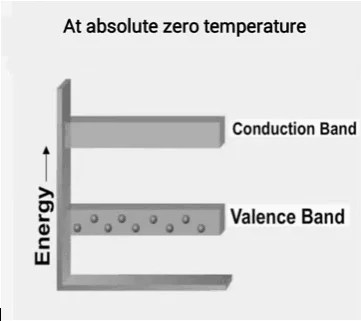Now at high temperature or by receiving some additional energy the electron from valence band jumps to the conduction band.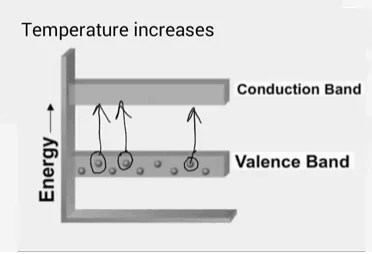As the electron jumps from the valence band to conduction band a vacancy is created in the valence band where the electron was initially present and this vacancy is known as a hole.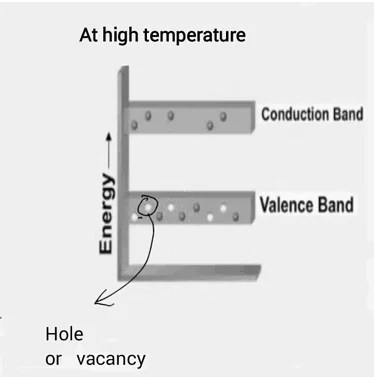Effective mass: The effective mass of a particle (denoted by m*)is the mass which it seems to have while responding to forces. This effective mass is given by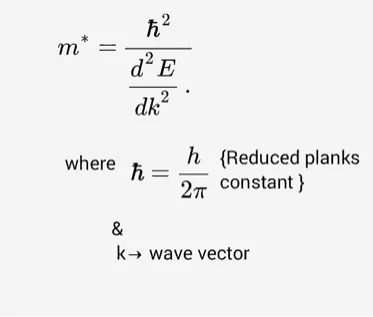## Intrinsic and extrinsic semiconductor

Semiconductors  are classified into two types

1. Intrinsic semiconductor
2. Extrinsic semiconductor

### Intrinsic semiconductor:

Intrinsic semiconductors are the semiconductors in a very pure form. Germanium and silicon are examples of intrinsic semiconductors. The crystalline structure  of Ge is shown :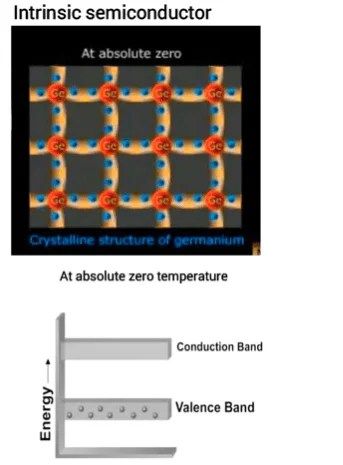At absolute zero temperature, all the inner electrons of a semiconductor are tightly held and the valence electrons are involved in covalent bonding and hence there is no free electron, so the crystal behaves as a perfect insulator at absolute zero temperature.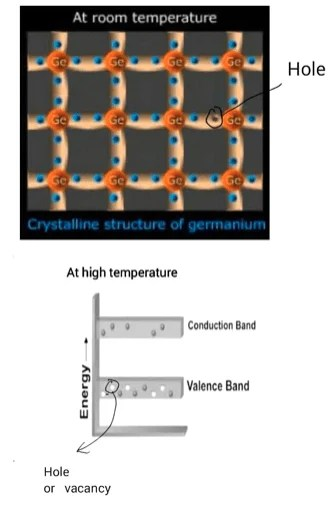Now at room temperature due to absorption of thermal energy, the Valence electrons get excited and jump from valence band to conduction band.  Now when a valence electron drift from the valence band to conduction band a vacancy is created, this vacancy is known as a hole. Thus an electron-hole pair is produced. Now is a potential difference is applied across the semiconductor current would flow through the circuit.

### Extrinsic semiconductor

Conductivity of intrinsic semiconductors is very low at room temperature. To increase the conductivity a small amount of external impurity is added to the intrinsic semiconductor. After the addition of an impurity, the semiconductor formed is known as an extrinsic semiconductor.

## Doping

The process of deliberately adding a small amount of impurity to a pure semiconductor to increase its conductivity is known as doping.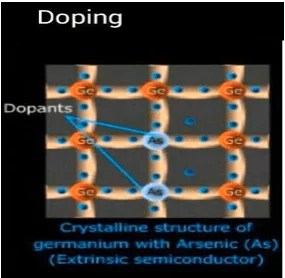## Dopants:

The impurities added in the process of doping are known as dopants.

Types of impurities or dopants

1. Donor impurities
2. Acceptor impurities

### Donor impurities

As the name suggests, the impurities which donate excess of electrons for conduction are called donor impurities.

Pentavalent elements or 5th group elements like antimony  (Sb), arsenic  (As) or phosphorus (k) are used as donor impurities. These impurities are also known as n-type impurities.

### Acceptor impurities

The impurities which create positive carriers or holes that can accept electrons for conduction are called acceptor impurities.

Trivalent elements or 3rd group elements like Boron, gallium or indium are generally used as acceptor impurities. These are also known as p-type impurities.

Depending upon the nature of impurity added the extrinsic semiconductor can be classified as:

1. N-type semiconductor
2. P-type semiconductor

## N-type semiconductor

If we dope a pure semiconductor with a Pentavalent or 5th group element an n-type semiconductor is formed.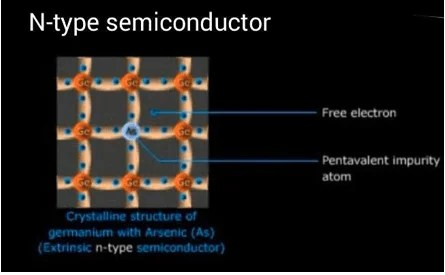As shown in fig. A Si atom is replaced by an arsenic atom. The arsenic atom has 5 valence electrons. As shown in fig 4 of these are involved in covalent bonding with the neighboring Si atoms but the 5th electron is free. As this electron is free and it moves, the arsenic atom gets a positive charge. But as arsenic is bound on all sides it cannot take part in conduction. Even though it is charged.

As on adding the dopant, we get a large concentration of free electrons or negative charge carriers the material is called n-type semiconductor (where n stands for negative ). Here the majority charge carriers are electrons and minority charge carriers are holes(thermally generated).

## P-type semiconductor

If we dope a pure semiconductor with a Trivalent or 3rd group element like Boron, gallium or indium which has 3 valence electrons a P-type semiconductor is formed.As shown in fig, if a Si atom is replaced by a gallium atom, gallium has 3 valence electrons and they are involved in covalent bonding with the neighboring Si atoms but there is a vacancy present at the 4th bond, this vacancy is a hole. Now to complete four covalent bonds it borrows an electron from the neighboring Si atom. As the electron moves from Si atom a hole is created at that atom which is replaced by another electron creating another hole. As the material has an excess of holes or positive charge carriers it is known as a P-type semiconductor. Here the holes are majority carriers while the electrons are minority carriers.

## Electron excitation

Excitation of an electron is the movement of an electron from a low energy level to a higher energy level. This can be done by using 2 types of energies. Light (photon energy) and thermal energy. This is known as photoexcitation and thermal excitation respectively.

### Photon excitation

In this type of excitation, electrons are excited by using photons. Here the electrons absorb energy from photons and jump to higher energy levels by acquiring this energy.

### Thermal excitation

Due to an increase in temperature of the surrounding the electron absorbs thermal energy and gets excited, this type of excitation is known as thermal excitation.

## Carriers concentration

### 1. Intrinsic semiconductor :

Due to the excitation of electrons from the valence band to conduction band, free carriers are created in both the bands. The concentration of these carriers is known as carriers concentration of intrinsic semiconductors.

The intrinsic carrier concentration is the number of electrons present in the conduction band or number of holes present in the valence band.

### 2. Extrinsic semiconductor :

In extrinsic semiconductors the concentration of carriers changes due to the addition of impurities. So, in an N-type semiconductor, electron concentration is more than the intrinsic concentration and hole concentration is less than the intrinsic concentration.

In a P-type semiconductor, the hole concentration is more than the intrinsic concentration and electron concentration is less than the intrinsic concentration.

Also at thermal equilibrium, the product of electron and hole concentration does not depend on the donor or acceptor doping.

It is given as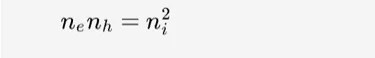For a P-type semiconductor if NA is the concentration of acceptor impurity atoms

as nh >>ne       ⇒ nh =~NA

electron concentration   (ne)For an N-type, if ND is the concentration of donor impurities atoms

as ne>>nh   ⇒ ne =~ND

holes concentration (nh)Related courses for this will be up soon!

This site uses Akismet to reduce spam. Learn how your comment data is processed.

Top